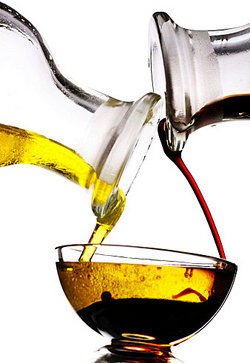# Examples of Density for Substances & in Real Life

Density is the measurement of how tightly or loosely a given substance is packed into a given volume. Air, for example, is low density, much lower than human tissue, which is why we can pass through it. The same does not apply to granite. Don’t try to walk through granite. It’s bad for you.Oil and vinegar being poured into a bowl as examples of density

Density is one of the basic properties of physical science. Physics, chemistry, and even biology require a clear understanding of what density is and how it’s calculated.

## Density Formula

Density is calculated according to the simple formula:

• p=M/v

That is, density (p) is equal to total mass (M) divided by total volume (v). This formula can be used to determine the density of any substance. Common units for the measurement of density include grams (g), milliliters (ml), or grams per cubic centimeter. For instance, if there are 50 grams of a given substance within a cubic centimeter, that unit’s density is 50g/mm3.

Density can vary according to temperature and pressure. Applying pressure to an object decreases volume, which then increases density. Increasing the temperature of an object almost always decreases its density by increasing its volume.

By heating the bottom of a volume, especially a fluid volume such as a liquid or gas, convection causes the substance to rise, thus increasing the volume. The temperature variation is typically minor in solids and most liquids, but much greater in gases. The abbreviations to know are:

• NTP: Normal Temperature and Pressure. Equal to 20 degrees C (68 F) and 1 atmosphere of pressure. Our examples below are all at NTP.
• STP: Standard Temperature and Pressure. Equal to 0 degrees C (32 F) and 1 atmosphere of pressure.

These standardized pressures and temperatures make it possible to confirm equal densities are being used regardless of the application.

## Everyday Density Examples

Examples of relative density, or different densities in different substances, occur throughout everyday life.

• In an oil spill in the ocean, the oil rises to the top because it is less dense than water, creating an oil slick on the surface of the ocean.
• A Styrofoam cup is less dense than a ceramic cup, so the Styrofoam cup will float in water and the ceramic cup will sink.
• Wood generally floats on water because it is less dense than water. Rocks, generally being denser than water, usually sink. This obvious example illustrates the power of science in real life. Many widely used hardwoods, such as ebony, mahogany and lignum vitae, are dense enough to sink in water, and a few rocks, such as pumice, are light enough to float. Scientifically, what counts isn’t the fact that one is wood and the other stone. All that matters is the relative densities of the substances.
• Helium balloons rise because helium is less dense than the surrounding air. Over time, the helium escapes the balloon and is replaced by air, causing it to sink.
• Oil floats on vinegar because its density is lower.

## Substances and Their Density

The following are examples of the density of various substances in grams per cubic centimeter, as measured at room temperature and 1 atmosphere of pressure (NTP).

### Gases

The density of gases is strongly affected by pressure and temperature. These have all been measured at room temperature and 1 atmosphere of pressure. Results are presented in gram per cubic centimeter.

 Substance Density(g/cm^3) Substance Density(g/cm^3) Air 0.001293 Methane 0.0006 Ammonia 0.000771 Neon 0.0009 Argon 0.0017 Nitrogen 0.00125 Carbon dioxide 0.00198 Nitrogen monoxide 0.001251 Carbon monoxide 0.00125 Nitrous oxide 0.0018 Chlorine 0.0032 Oxygen 0.00143 Fluorine 0.0017 Ozone 0.0021 Helium 0.00018 Radon 0.01 Hydrogen 0.00009 Sulphur monoxide 0.003 Krypton 0.0037 Xenon 0.0053

### Liquids

These substances are liquid at room temperature. As a rule, they are less affected by pressure and temperature than gases, but there are still measurable differences. These are their densities by gram per cubic centimeter at room temperature and 1 atmosphere of pressure.

 Substance Density(g/cm^3) Substance Density(g/cm^3) Acetic acid (vinegar) 1.049 Kerosene 0.201 Ammonia 0.771 Mercury 13.593 Butane 0.599 Methanol 0.791 Citric acid 1.660 Nitric acid 1.420 Corn syrup 38.000 Octane 0.699 Crude oil 0.790 Paraffin 0.800 Ether 0.714 Propane 0.494 Gasoline 0.749 Turpentine 0.868 Glycerine 1.259 Water 1.000 Isopropyl alcohol 0.786 Whale oil 0.925